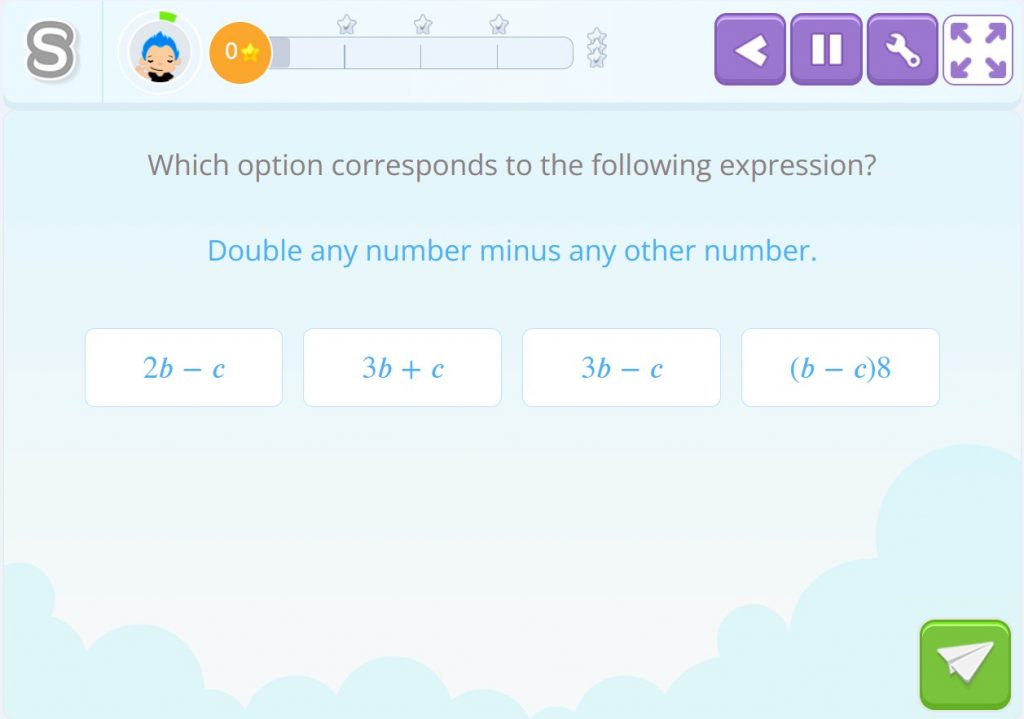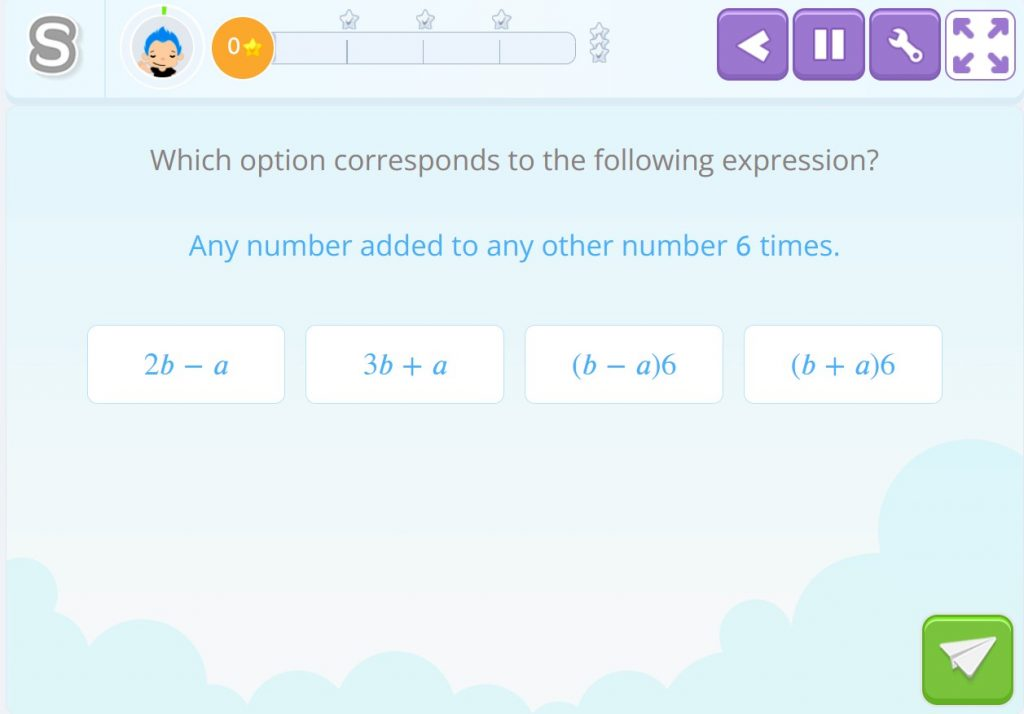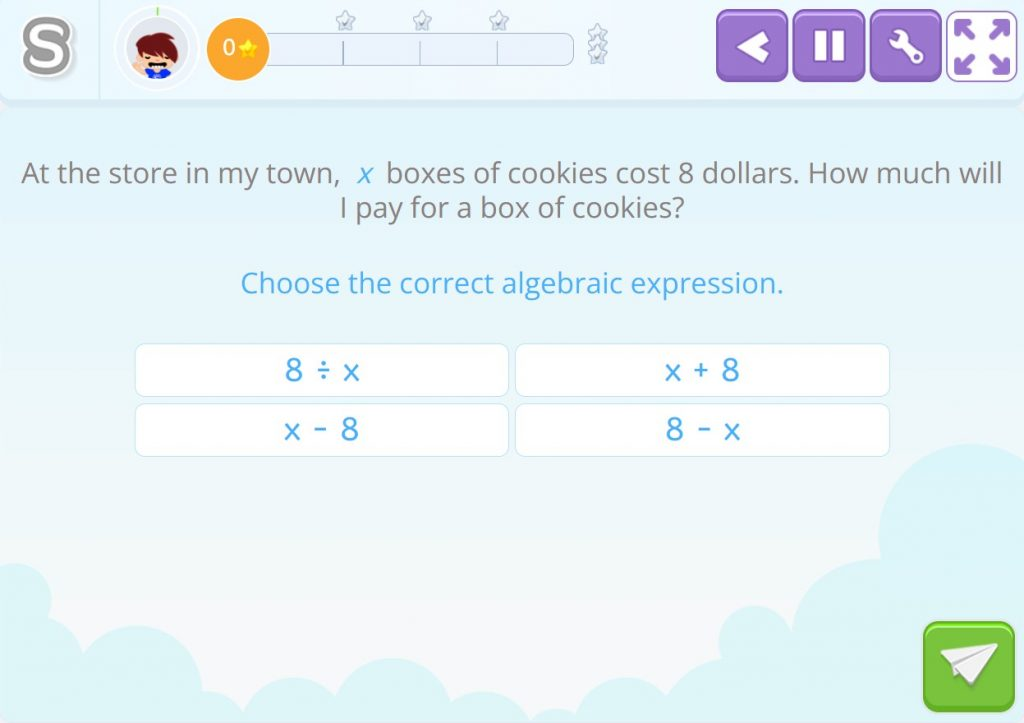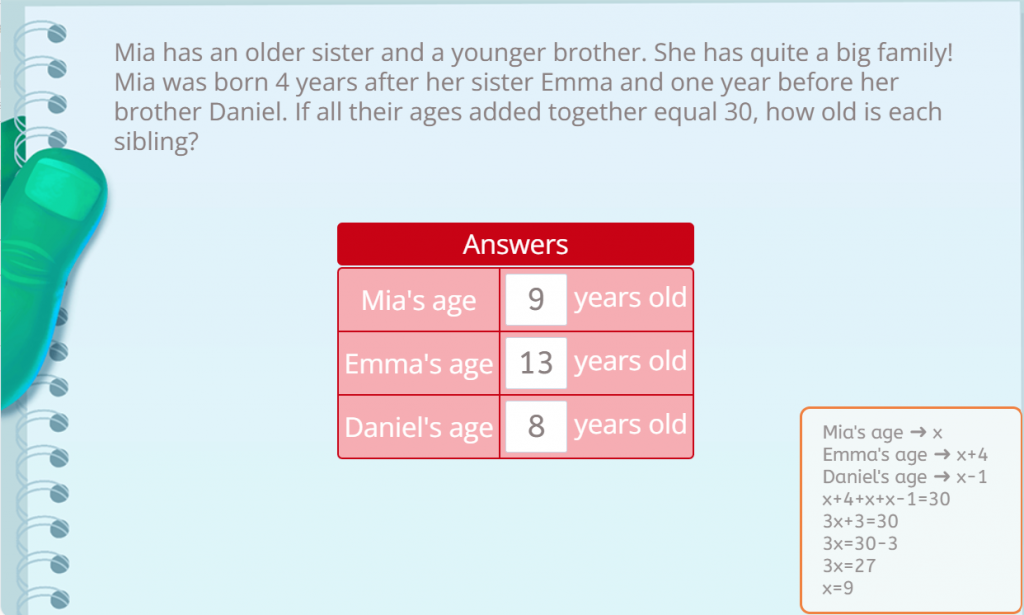Smartick is a fun way to learn math!Mar24

# Algebra Problems: 3 Types of Activities in Smartick

Algebra is a branch of mathematics that focuses on the study of mathematical structures and the operations that can be performed with them. In particular, algebra focuses on the use of symbols and letters to represent numbers and mathematical expressions, making it possible to work with them in a general and abstract way.

In algebra, letters and symbols are used to represent variables and constants, and operations such as addition, subtraction, multiplication and division are performed with them. In this post, we are going to see some examples of algebra problems that are posed in Smartick.

Index

## How to solve algebra problems?

Solving algebra problems may seem difficult at first, but with practice and an understanding of some basic concepts, it can be easier than you might think. Here are some steps you can take to solve algebra problems:

1. Understand the question: The first thing you should do is read the question and make sure you understand what is being asked. If you do not understand the question, you are likely to have difficulty solving the problem.
2. Identify what is known: After you understand the question, review the data provided and write down everything you know about the problem.
3. Identify what needs to be found: Determine what you are being asked to find. This will help you know what operations you need to perform to solve the problem.
4. Translate the information into algebraic expressions: Convert the information provided into algebraic expressions. Use variables to represent unknown quantities and mathematical symbols to represent operations.
5. Solve the equation: Use appropriate mathematical tools to solve the equation. This may include simplifying expressions, using algebraic properties, and solving linear or quadratic equations.
6. Check the solution: Once you have found the solution, verify that the answer is reasonable and meets the requirements of the problem.

## Examples of algebra problems in Smartick

When we encounter an algebra problem, we have to follow the steps explained above. It is very important to express the unknown data or data with an unknown or letter, such as “x”. This data will be the one we have to solve or find.

There are many different types of algebra problems, where we have to find a number or solve a problem of ages or money. Each of these algebra problems are solved differently because each of them can have a specific algebraic expression.

In the Smartick sessions, we work on several types of algebra problems. Here there are some examples.

### Translate the information

With these types of algebra problems, we apply step 4 mentioned in the previous section: convert the information provided into algebraic expressions. Use variables to represent unknown quantities and mathematical symbols to represent operations.

• Example 1:Double any number minus any other number corresponds to the following algebraic expression:

2b – c

• Example 2:Any number added to any other number 6 times corresponds to the following algebraic expression:

(b + a)6

### Translate the information into a problemYou have to divide 8 dollars by “x” boxes of cookies, so the correct answer is:

8 ÷ x

### Age-related equation problemsIn this case we have to find the value of the age of Mia, Emma and Daniel.

The data of the problem are:

• Mia was born 4 years later than her sister Emma and one year before her brother Daniel:
• Mia’s age → x
• Emma’s age → x+4
• Daniel’s age → x-1
• We are asked: How old is each of them?

The first degree equation that solves this equation problem is: x+4+x+x+x-1=30. The value of x is 9. Therefore, Mia is 9 years old, Emma is 13 years old, and Daniel is 8 years old.

Finally, if you want, you can take a look at these posts that may be of interest to you:

And if you want to keep practicing more content like this and learning elementary school maths, sign up for Smartick.